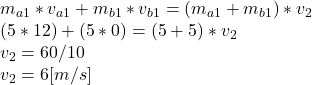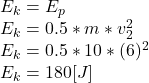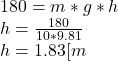## A 5 kg chunk of ice is sliding at 12 m/s on the frictionless floor of an ice-covered valley when it collides with and sticks to

Question

A 5 kg chunk of ice is sliding at 12 m/s on the frictionless floor of an ice-covered valley when it collides with and sticks to another 5 kg
chunk of ice that is initially at rest as shown. After the collision, how high above the valley floor will the combined chunks go?

in progress 0
1 week 2021-09-05T09:13:38+00:00 1 Answers 0 views 0

The elevation will be 1.83 [m]

Explanation:

This problem can be solved by two stages, the first is to analyze the conservation of the moment, then by means of the conservation of energy find the height that both bodies can rise.

For the conservation of momentum, we know that the sum of the products of the masses by the speeds before the collision should be equal to the product of the masses by the speeds after the collision. The key here is to know that after the collision the mass will be equal to the sum of both bodies and that they will have a single speed at the end, this by means of the momentum conservation law can be represented below

ma1 = 5 [kg]

mb1 = 5 [kg]

va1 = 12 [m/s]

vb1 = 0

m2 = ma1 + mb1 = 5 + 5 = 10 [kg]

therefore:Now we can find the kinetic energy at that time with the calculated speed.By the conservation of the energy, we know that the energy will be transformed from kinetics to potential energy, therefore we can find the height that will reach with that energy.